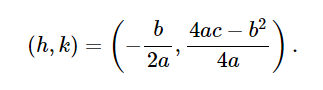## When Helping Means Winning contest

Ask a school-related question in the Help Zone and you could win a \$1000 scholarship!

# Help Zone

### Student Question

It's fair to convert vertex form to general form and general form to vertex form and so I need help to change from

general form to standard form, In joint, there is an illustration.

Mathematics## Explanations (1)

•Explanation from AlloprofExplanation from Alloprof

This Explanation was submitted by a member of the Alloprof team.

Hi ExtraordinaryMelon4228,

Before starting the resolution, we need to understand the different form of quadratic functions. The general form is this one:

$$f(x)=ax^2+bx+c$$

In this problem, we want the standard form, which is this one:

$$f(x)=a(x-h)^2+k$$

To go from the general form to the standard form, we need to use this formula:We then have the values of h and k, we can now write the function in the standard form. You can also use the completing square technique, it's just a bit longer to do.

I hope that helps and feel free to write us again!

Anthony B.